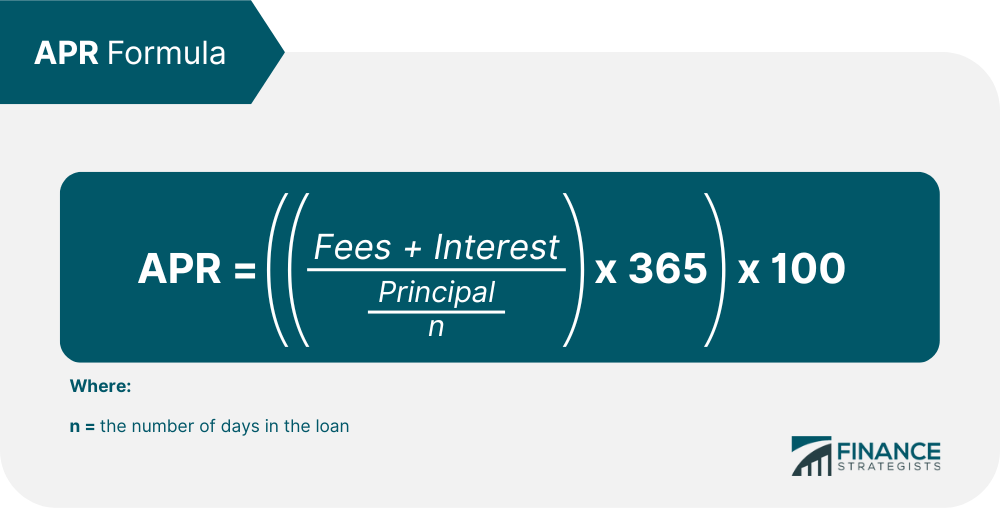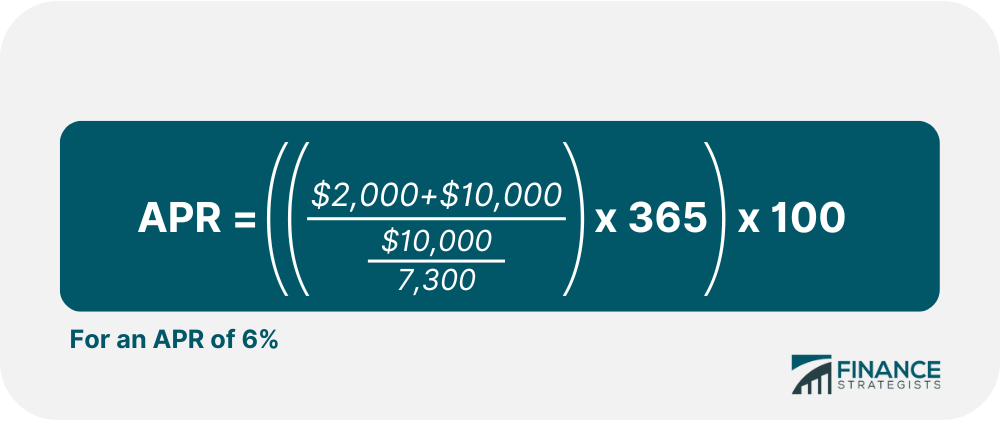# Interest Rate### Written byTrue Tamplin, BSc, CEPF® | Reviewed by Editorial Team

Updated on December 10, 2022

## Define Interest Rate in Simple Terms

The interest rate is the amount a lender charges a borrower for the use of assets.

The assets borrowed could be cash, such as in the case of a loan, but could also be consumer goods or physical assets such as a vehicle or property.

The interest rate is expressed as a percentage of the principal amount borrowed.

It is, in essence, a rental charge; in the case of large assets like vehicles or real estate, the leasing charge may serve as the interest rate.

## How Does an Interest Rate Work?

A country's central bank establishes a benchmark interest rate, which guides other banks when they decide what interest rate ranges they offer.

Banks will charge some sort of premium to this rate when making loans to consumers and businesses.

The overall state of the economy is a main factor in central bank policy.

Individual interest rates are also affected by the credit quality of the borrower.

If the borrower has a poor credit history, they may be considered "high-risk,"and if granted a loan, the interest they pay will be charged at a higher level commensurate with the credit worthiness of the borrower.

The opposite is true of borrowers with good credit quality; the interest on their loans may be less than average.

Interest rates tend to rise with inflation. When interest rates are high, it means that the cost of debt is high as well, which slows consumer demand for loans.

To combat inflation, central banks sometimes increase interest rates to reduce the overall money supply in an economy.

When the cost of borrowing is high, it discourages consumers from taking out expensive loans. This causes spending to slow down, which in turn causes prices to drop, and slows down inflation.

## How Interest Is Calculated

The most basic kind of interest is simple, or nominal, interest.

When a loan uses simple interest, it means that the percentage of the principal, the interest rate, gets added to the loan balance once per period.

For example, say an individual takes out a bank loan of \$10,000 at 5% interest.

The loan has a length of 20 years. Every year, 5% of \$10,000 will be added to the balance. After the first year, the new balance will be \$10,500; after two, the balance will be \$11,000, and so one. By year twenty, the balance will be \$10,000 + (\$500 * 20), or \$20,000.

However, the nominal interest rate on a loan doesn't always tell the whole story. Often, especially in the case of consumer loans, the interest rate of a loan is expressed as APR.

APR, or Annual Percentage Rate, uses simple interest to determine a rate of payment, but it takes into account all fees that are charged on the principal of the loan, such as closing costs, origination fees, and so on.

Let's say that the lender of the above loan charges fees totalling \$2,000 at 5% interest.

To determine the APR, use the formula:In this case, the equation looks like this:Sometimes interest can also be compounding.

This means that every time the interest rate is applied, it is applied to the current loan balance instead of the base principal.

After one year in a compounding environment, the above loan would still be \$10,500; after two, the interest rate would take 5% of \$10,500, for a new balance of \$11,025.

When a loan charges fees and the interest is compounding, the most accurate interest rate to look at is the EAR.

EAR, which stands for Effective Annual Rate, is the same as APY, or Annual Percentage Yield.

Knowing the APR of a loan offers a shortcut in calculating the EAR; in this case, just consider the \$10,000 loan to be compounding yearly at 6% rather than 5%.

Using the equation \$10,000*[(1+0.06)20-1] gets \$10,000 * 2.21 or \$22,100.

Added on to the principal gets a final amount due of \$32,100.

Interest rates are one of the most important aspects in every economic system. Examine how interest rates provide insight into future economic and financial market activity. Connect with a financial advisor in Jefferson County, KY or visit our financial advisor page to connect with one closest to your area.

## Interest Rate Definition FAQs

### What is an Interest Rate?

The interest rate is the amount a lender charges a borrower for the use of assets. The interest rate is expressed as a percentage of the principal amount borrowed.

### How does Interest Rate work?

A country’s central bank establishes a benchmark interest rate, which guides other banks when they decide what interest rate ranges they offer.

### How do Interest Rates change for individual borrowers?

Individual interest rates are also affected by the credit quality of the borrower. If the borrower has a poor credit history, they may be considered “high-risk,” and if granted a loan, the interest they pay will be charged at a higher level commensurate with the credit worthiness of the borrower.

### How is Interest Rate calculated?

When a loan uses simple interest, it means that the percentage of the principal, the interest rate, gets added to the loan balance once per period.

### How does raising Interest Rates slow down inflation?

To combat inflation, central banks sometimes increase interest rates to reduce the overall money supply. When the cost of borrowing is high, it discourages consumers from taking out expensive loans, which slows spending, which in turn causes prices to drop, and slows down inflation.